# NCERT Solutions for Class 7 Maths Chapter 6 The Triangle and its Properties (EX 6.5) Exercise 6.5

## NCERT Solutions for Class 7 Maths Chapter 6 The Triangle and its Properties (EX 6.5) Exercise 6.5

Free PDF download of NCERT Solutions for Class 7 Maths Chapter 6 Exercise 6.5 (EX 6.5) and all chapter exercises at one place prepared by expert teacher as per NCERT (CBSE) books guidelines. Class 7 Maths Chapter 6 The Triangle and its Properties Exercise 6.5 Questions with Solutions to help you to revise complete Syllabus and Score More marks. Register and get all exercise solutions in your emails. Every NCERT Solution is provided to make the study simple and interesting on Vedantu. Subjects like Science, Maths, English will become easy to study if you have access to NCERT Solution for Class 7 Science , Maths solutions and solutions of other subjects.

Do you need help with your Homework? Are you preparing for Exams?
Study without Internet (Offline)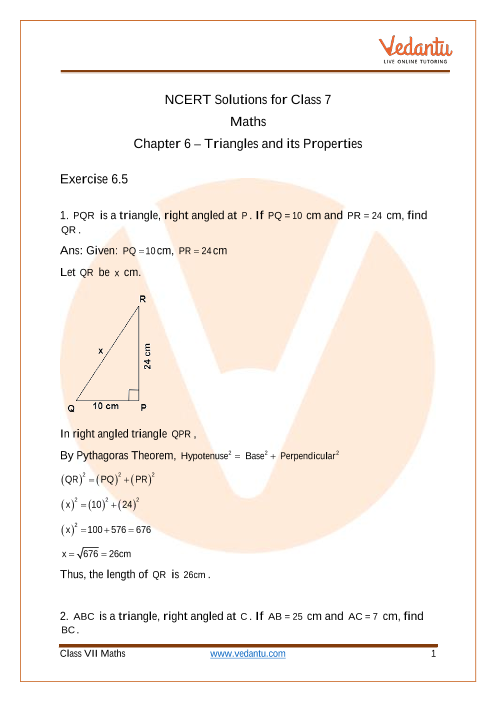Book your Free Demo session
Get a flavour of LIVE classes here at VedantuVedantu Improvement Promise
We promise improvement in marks or get your fees back. T&C Apply*

## Access NCERT Solutions for Class 7 Chapter 6- Triangles and its Properties

Exercise 6.5

1. $PQR$ is a triangle, right angled at $P$. If $PQ = 10$cm and $PR = 24$cm, find $QR$.

Ans: Given: $PQ = 10$cm, $PR = 24$cm

Let $QR$ be $x$cm.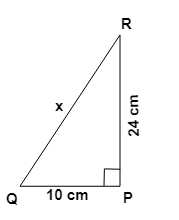In right angled triangle $QPR$,

By Pythagoras Theorem, ${\text{Hypotenus}}{{\text{e}}^2} = {\text{ Bas}}{{\text{e}}^2} + {\text{ Perpendicula}}{{\text{r}}^2}$

${\left( {QR} \right)^2} = {\left( {PQ} \right)^2} + {\left( {PR} \right)^2}$

${\left( x \right)^2} = {\left( {10} \right)^2} + {\left( {24} \right)^2}$

${\left( x \right)^2} = 100 + 576 = 676$

$x = \sqrt {676} = 26cm$

Thus, the length of  $QR$ is $26cm$.

2. $ABC$ is a triangle, right angled at $C$. If $AB = 25$cm and $AC = 7$cm, find $BC$.

Ans: Given: $AB = 25$cm, $AC = 7$cm

Let $BC$ be $x$cm.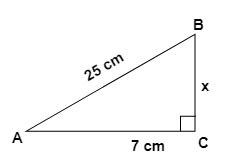In right angled triangle $ACB$,

By Pythagoras Theorem, ${\text{Hypotenus}}{{\text{e}}^2} = {\text{ Bas}}{{\text{e}}^2} + {\text{ Perpendicula}}{{\text{r}}^2}$

${\left( {AB} \right)^2} = {\left( {AC} \right)^2} + {\left( {BC} \right)^2}$

${\left( {25} \right)^2} = {\left( 7 \right)^2} + {\left( x \right)^2}$

$625 = 49 + {x^2}$

${x^2} = 625 - 49 = 576$

$x = \sqrt {576} = 24cm$

Thus, the length of  $BC$is $24cm$.

3. A $15$m long ladder reached a window $12$m high from the ground on placing it against a wall at a distance $a$. Find the distance of the foot of the ladder from the wall.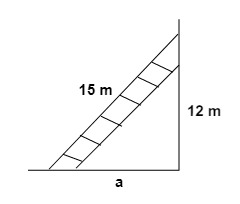Ans: Let $AC$ be the ladder and $A$ be the window.

Given: $AC = 15$m, $AB = 12$m

Let $CB$ be $a$m.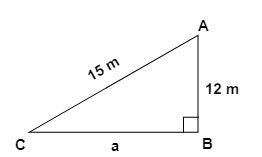In right angled triangle $ACB$,

By Pythagoras Theorem, ${\text{Hypotenus}}{{\text{e}}^2} = {\text{ Bas}}{{\text{e}}^2} + {\text{ Perpendicula}}{{\text{r}}^2}$

${\left( {AC} \right)^2} = {\left( {CB} \right)^2} + {\left( {AB} \right)^2}$

${\left( {15} \right)^2} = {\left( {12} \right)^2} + {\left( a \right)^2}$

$225 = {a^2} + 144$

${a^2} = 225 - 144 = 81$

$x = \sqrt {81} = 9m$

Hence, the distance of the foot of the ladder from the wall is $9m$.

4. Which of the following can be the sides of a right triangle? In the case of right angled triangles, identify the right angles.

(i) $2.5$cm, $6.5$cm, $6$cm

Ans: Let us consider, the larger side be the hypotenuse and also using Pythagoras theorem,

${\text{Hypotenus}}{{\text{e}}^2} = {\text{ Bas}}{{\text{e}}^2} + {\text{ Perpendicula}}{{\text{r}}^2}$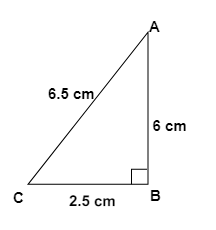In $\vartriangle ABC$,               ${\left( {AC} \right)^2} = {\left( {AB} \right)^2} + {\left( {BC} \right)^2}$

$L.H.S. = {\left( {6.5} \right)^2} = 42.25cm$

$R.H.S. = {\left( 6 \right)^2} + {\left( {2.5} \right)^2} = 36 + 6.25 = 42.25cm$

Since, $L.H.S. = R.H.S.$

Therefore, the given sides form a right angled triangle.

Right angle lies on the opposite to the greater side $6.5$cm, i.e., at $B$.

(ii) $2$cm, $2$cm, $5$cm

Ans: Let us consider, the larger side be the hypotenuse and also using Pythagoras theorem,

${\text{Hypotenus}}{{\text{e}}^2} = {\text{ Bas}}{{\text{e}}^2} + {\text{ Perpendicula}}{{\text{r}}^2}$

${\left( 5 \right)^2} = {\left( 2 \right)^2} + {\left( 2 \right)^2}$

$L.H.S. = {\left( 5 \right)^2} = 25cm$

$R.H.S. = {\left( 2 \right)^2} + {\left( 2 \right)^2} = 4 + 4 = 8cm$

Since, $L.H.S. \ne R.H.S.$

Therefore, the given sides do not form a right angled triangle.

(iii) $1.5$cm, $2$cm, $2.5$cm

Ans: Let us consider, the larger side be the hypotenuse and also using Pythagoras theorem,

${\text{Hypotenus}}{{\text{e}}^2} = {\text{ Bas}}{{\text{e}}^2} + {\text{ Perpendicula}}{{\text{r}}^2}$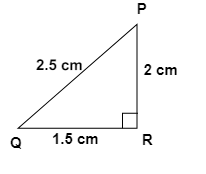In $\vartriangle PQR$,               ${\left( {\operatorname{P} R} \right)^2} = {\left( {PQ} \right)^2} + {\left( {RQ} \right)^2}$

$L.H.S. = {\left( {2.5} \right)^2} = 6.25cm$

$R.H.S. = {\left( 2 \right)^2} + {\left( {1.5} \right)^2} = 4 + 2.25 = 6.25cm$

Since, $L.H.S. = R.H.S.$

Therefore, the given sides form a right angled triangle.

Right angle lies on the opposite to the greater side $2.5$cm, i.e., at $Q$.

5. A tree is broken at a height of $5$m from the ground and its top touches the ground at a distance of $12$m from the base of the tree. Find the original height of the tree.

Ans: Let $A'CB$ represents the tree before it broken at the point $C$ and let the

top $A'$ touches the ground at $A$ after it broke. Then $\vartriangle ABC$ is a right angled triangle, right angled at $B$.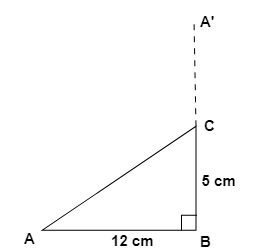$AB = 12$m and $BC = 5$m

Using Pythagoras theorem, In $\vartriangle ABC$

${\left( {AC} \right)^2} = {\left( {AB} \right)^2} + {\left( {BC} \right)^2}$

${\left( {AC} \right)^2} = {\left( {12} \right)^2} + {\left( 5 \right)^2}$

${\left( {AC} \right)^2} = 144 + 25$

${\left( {AC} \right)^2} = 169$

$AC = 13m$

The total height of the tree is sum of sides $AC$ and $CB$ that is$AC + CB = 13 + 5 = 18m$.

6. Angles $Q$ and $R$ of a $\vartriangle PQR$ are ${25^ \circ }$ and ${65^ \circ }$.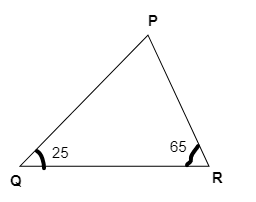Write which of the following is true:

(i) $P{Q^2} + Q{R^2} = R{P^2}$

(ii) $P{Q^2} + R{P^2} = Q{R^2}$

(iii) $R{P^2} + Q{R^2} = P{Q^2}$

Ans: In $\vartriangle PQR$,

$\angle PQR + \angle QRP + \angle RPQ = {180^ \circ }$                 By Angle sum property of a [$\vartriangle$]

${25^ \circ } + {65^ \circ } + \angle RPQ = {180^ \circ }$              $\Rightarrow$          ${90^ \circ } + \angle RPQ = {180^ \circ }$

$\angle RPQ = {180^ \circ } - {90^ \circ } = {90^ \circ }$

Thus, $\vartriangle PQR$ is a right angled triangle, right angled at $P$.

$\therefore {\text{ Hypotenus}}{{\text{e}}^2} = {\text{ Bas}}{{\text{e}}^2} + {\text{ Perpendicula}}{{\text{r}}^2}$       (By Pythagoras theorem)

$Q{R^2} = P{Q^2} + R{P^2}$

Hence, Option (ii) is correct.

7. Find the perimeter of the rectangle whose length is $40$cm and a diagonal is $41$cm.

Ans: Given diagonal $PR = 41$cm, length $PR = 40$cm

Let breadth $\left( {QR} \right)$ be $x$cm.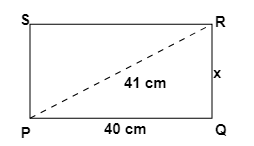Now, in right angled triangle $PQR$,

${\left( {PR} \right)^2} = {\left( {RQ} \right)^2} + {\left( {PQ} \right)^2}$                         (By Pythagoras theorem)

${\left( {41} \right)^2} = {\left( x \right)^2} + {\left( {40} \right)^2}$

$1681 = {\left( x \right)^2} + 1600$

${x^2} = 1681 - 1600$

${x^2} = 81$

$x = \sqrt {81} = 9cm$

Therefore the breadth of the rectangle is $9$cm.

${\text{Perimeter of rectangle = 2}}\left( {{\text{length + breadth}}} \right)$

$= 2\left( {9 + 49} \right)$

$= 2 \times 49 = 98cm$

Hence the perimeter of the rectangle is $98$cm.

8. The diagonals of a rhombus measure $16$cm and $30$cm. Find its perimeter.

Ans: Given: Diagonals $AC = 30$cm and $DB = 16$cm.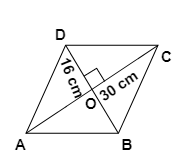Since the diagonals of the rhombus bisect at right angle to each other so it divides the diagonals into equal halves.

Therefore, $OD = \frac{{DB}}{2} = \frac{{16}}{2} = 8cm$

And            $OC = \frac{{AC}}{2} = \frac{{30}}{2} = 15cm$

Now, In right angle triangle $DOC$,

${\left( {DC} \right)^2} = {\left( {OD} \right)^2} + {\left( {OC} \right)^2}$                                   (By Pythagoras theorem)

${\left( {DC} \right)^2} = {\left( 8 \right)^2} + {\left( {15} \right)^2}$

${\left( {DC} \right)^2} = 64 + 225 = 289$

$DC = \sqrt {289} = 17cm$

${\text{Perimeter of rhombus = }}4 \times {\text{Side}}$

$= 4 \times 17 = 68cm$

Thus, the perimeter of rhombus is $68$ cm.

## NCERT Solutions For Class 7 Maths Chapter 6 The Triangle and its Properties Exercise 6.5

Opting for the NCERT solutions for Ex 6.5 Class 7 Maths is considered as the best option for the CBSE students when it comes to exam preparation. This chapter consists of many exercises. Out of which we have provided the Exercise 6.5 Class 7 Maths NCERT solutions on this page in PDF format. You can download this solution as per your convenience or you can study it directly from our website/ app online.

Vedantu in-house subject matter experts have solved the problems/ questions from the exercise with the utmost care and by following all the guidelines by CBSE. Class 7 students who are thorough with all the concepts from the Maths textbook and quite well-versed with all the problems from the exercises given in it, then any student can easily score the highest possible marks in the final exam. With the help of this Class 7 Maths Chapter 6 Exercise 6.5 solutions, students can easily understand the pattern of questions that can be asked in the exam from this chapter and also learn the marks weightage of the chapter. So that they can prepare themselves accordingly for the final exam.

Besides these NCERT solutions for Class 7 Maths Chapter 6 Exercise 6.5, there are plenty of exercises in this chapter which contain innumerable questions as well. All these questions are solved/answered by our in-house subject experts as mentioned earlier. Hence all of these are bound to be of superior quality and anyone can refer to these during the time of exam preparation. In order to score the best possible marks in the class, it is really important to understand all the concepts of the textbooks and solve the problems from the exercises given next to it.

Do not delay any more. Download the NCERT solutions for Class 7 Maths Chapter 6 Exercise 6.5 from Vedantu website now for better exam preparation. If you have the Vedantu app in your phone, you can download the same through the app as well. The best part of these solutions is these can be accessed both online and offline as well.SHARETWEETSHARESUBSCRIBE